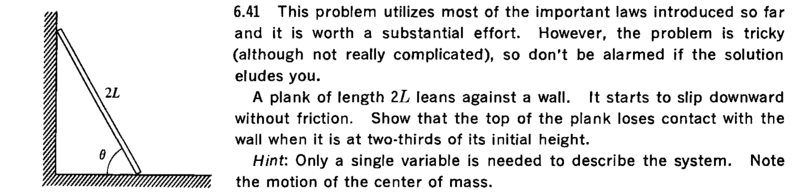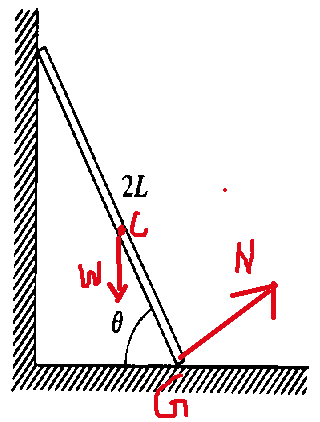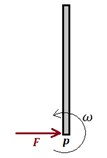# At what height does this plank leave the wall

## Homework Statement## The Attempt at a SolutionWhen the plank leavs the wall at angle ##\theta ##,

Torque about ground ## \tau _g = mgl \cos \theta = \frac { m(2l)^2 \alpha }3##
Torque about COM ## \tau _c = Nl =\frac { m(2l)^2 \alpha } {12} ##
Acceleration of COM: a = ## [g^2 +( \frac N m )^2 - 2 g \frac N m \cos \theta]^{0.5} = \alpha l ##

Is this correct so far?
I think the last eqn. is wrong as further calculation gives ##\cos \theta =1##

Last edited:

robphy
Homework Helper
Gold Member

Yes, it is.
The wall could not exert any force as the plank leaves it.
So, there are only two forces acting on it which I have shown already.

mfb
Mentor
What is ##\alpha## and where does it come from in the torque equation? There is also the motion of the center of mass to consider.

What is αα\alpha and where does it come from in the torque equation?
##\alpha ## is the standard symbol used for angular acceleration. It is in the torque equation.
There is also the motion of the center of mass to consider.
The last eqn in the OP is about the above.

mfb
Mentor
##\alpha ## is the standard symbol used for angular acceleration.
I know, but it doesn't seem to make sense at this place because you do not have a pure rotation.

SammyS
Staff Emeritus
Homework Helper
Gold Member

## Homework Statement

View attachment 209084

## The Attempt at a Solution

View attachment 209085
When the plank leaves the wall at angle ##\theta ##,

Torque about ground ## \tau _g = mgl \cos \theta = \frac { m(2l)^2 \alpha }3##
Torque about COM ## \tau _c = Nl =\frac { m(2l)^2 \alpha } {12} ##
Acceleration of COM: a = ## [g^2 +( \frac N m )^2 - 2 g \frac N m \cos \theta]^{0.5} = \alpha l ##

Is this correct so far?
I think the last eqn. is wrong as further calculation gives ##\cos \theta =1##
Normal force is perpendicular to the surface. You have the orientation of ##\ \vec N \ ## wrong in your FBD .

Does the wall (vertical) also provide a normal force?

There is more to the motion of the COM than simply giving its acceleration.

Chestermiller
Mentor
In terms of L and ##\theta##, what are the coordinates of the center of mass? In terms of these kinematic parameters, what are the velocity components of the center of mass? What are the acceleration components of the center of mass? What are the force balances in the vertical and horizontal directions?

Chestermiller
Mentor
Calculus is not needed.
Hummmm. Sound interesting.

haruspex
Homework Helper
Gold Member
2020 Award
Hummmm. Sound interesting.
You can obtain the velocity and angular velocity as functions of the initial and current angles just from conservation. It follows that it can be solved by considering the instantaneous circumstances at the current angle, since no further history can be relevant.

•mfb and Chestermiller
TSny
Homework Helper
Gold Member
You can obtain the velocity and angular velocity as functions of the initial and current angles just from conservation. It follows that it can be solved by considering the instantaneous circumstances at the current angle, since no further history can be relevant.
Yes. If you are willing to take one time derivative, you can get the needed info about angular acceleration from the energy equation. No need to set up torque or force equations (other than knowing that zero horizontal force implies zero horizontal acceleration of cm). I think we each have our own way that we like to solve this problem.•ehild
haruspex
Homework Helper
Gold Member
2020 Award
If you are willing to take one time derivative
Yes. Or to avoid calculus entirely, take moments about the instantaneous centre of rotation.

•Nidum
Chestermiller
Mentor
Yes. Or to avoid calculus entirely, take moments about the instantaneous centre of rotation.
I'm intrigued by this. I was able to solve this problem using conservation of energy in conjunction with the force balance in the x direction, but had to use calculus to express the linear velocity- and acceleration components of the center of mass in terms of the angular velocity and angular acceleration. Please share how you were able to solve without using calculus.

haruspex
Homework Helper
Gold Member
2020 Award
I'm intrigued by this. I was able to solve this problem using conservation of energy in conjunction with the force balance in the x direction, but had to use calculus to express the linear velocity- and acceleration components of the center of mass in terms of the angular velocity and angular acceleration. Please share how you were able to solve without using calculus.
Did you identify the instantaneous centre of rotation?

Normal force is perpendicular to the surface. You have the orientation of ## \vec N##wrong in your FBD .
There are two surfaces and so there are two normal to the surfaces, 1) normal to the ground 2) normal to the plank?
Since you said that the one I took was wrong , I think ##\vec N ## will be normal to the ground. But, in general how to decide the correct normal?

Does the wall (vertical) also provide a normal force?
No, it doesn't, as when the plank leaves the wall, it loses contact with the wall. So, at the moment of plank leaving the wall, I have to take the normal force exerted by the wall to be 0.

About other posts, I will reply after digesting them. Thanks for them.

Chestermiller
Mentor
Did you identify the instantaneous centre of rotation?
Is that the contact point on the horizontal surface?

haruspex
Homework Helper
Gold Member
2020 Award
Is that the contact point on the horizontal surface?
No.
At each instant, each point of the plank will be moving tangentially to the instantaneous rotation centre. So just take the normals to the contact points and see where they intersect.

•mfb
Chestermiller
Mentor
No.
At each instant, each point of the plank will be moving tangentially to the instantaneous rotation centre. So just take the normals to the contact points and see where they intersect.
This is new to me. I'll have to think about it.

TSny
Homework Helper
Gold Member
Yes. Or to avoid calculus entirely, take moments about the instantaneous centre of rotation.
By taking moments about the instantaneous center of rotation, do you mean setting up the equation ##\sum \tau_p = I_p \alpha##, where ##p## denotes the instantaneous center of rotation? This equation is not true in general, but it does happen to be true for this problem. Its validity here was not obvious to me until I justified it. Maybe I'm overlooking an easy way to see that it's true in this problem.

but it doesn't seem to make sense at this place because you do not have a pure rotation.
Yes, but the motion could be divided into pure rotation and pure translation. So, I think a ≠ αl, but are other parts of the three eqns correct in OP?

haruspex
Homework Helper
Gold Member
2020 Award
This equation is not true in general,
Actually, it is.
As I was taught at school, it is ok to use any fixed point in an inertial frame, the mass centre of the object, or the instantaneous centre of rotation of the object.
At the time, it bothered me that it was so special-case. Recently I investigated this and found that the set of points of the object which, taken as axis, happen to give the right answer form a circle passing through the mass centre and the instantaneous centre of rotation.

TSny
Homework Helper
Gold Member
I can see why ##\sum \tau_p = dL_p/dt## is valid. It's the equating of ##dL_p/dt## with ##I_p \alpha## that I don't think is always valid.

For example, suppose at some instant of time a rod is instantaneously rotating about one end, p, with angular speed ##\omega##. Also, at this instant there is a single force F applied to the rod at p, as shown. Then ##\sum \tau_p = dL_p/dt## is valid since you can show that both sides are zero. But ##\alpha \neq 0##. So, ##\sum \tau_p \neq I_p \alpha##.TSny
Homework Helper
Gold Member
In that model, the linear acceleration of the mass centre has moment about the chosen axis. That is all part of the rate of change of angular momentum.
Yes. I think maybe I've misinterpreted what you were originally saying when you said "take moments about the instantaneous center of rotation". If you meant set up ##\sum \tau_p = dL_p/dt## and then show that ##dL_p/dt = I_p \alpha##, then I'm with you. But if a student were to start with ##\sum \tau_p = I_p \alpha##, I would ask for justification since I don't think this equation is generally valid.